# NCERT Solutions For Class 10 Maths Chapter 14 Statistics Ex 14.2

Get Free NCERT Solutions for Class 10 Maths Chapter 14 Ex 14.2 PDF. Statistics Class 10 Maths NCERT Solutions are extremely helpful while doing your homework. Exercise 14.2 Class 10 Maths NCERT Solutions were prepared by Experienced ncert-books.in Teachers. Detailed answers of all the questions in Chapter 14 Maths Class 10 Statistics Exercise 14.2 provided in NCERT TextBook.

Question 1.
The following table shows the ages of the patients admitted in a hospital during a year.Find the mode and the mean of the data given above. Compare and interpret the two measures of central tendency.
Solution: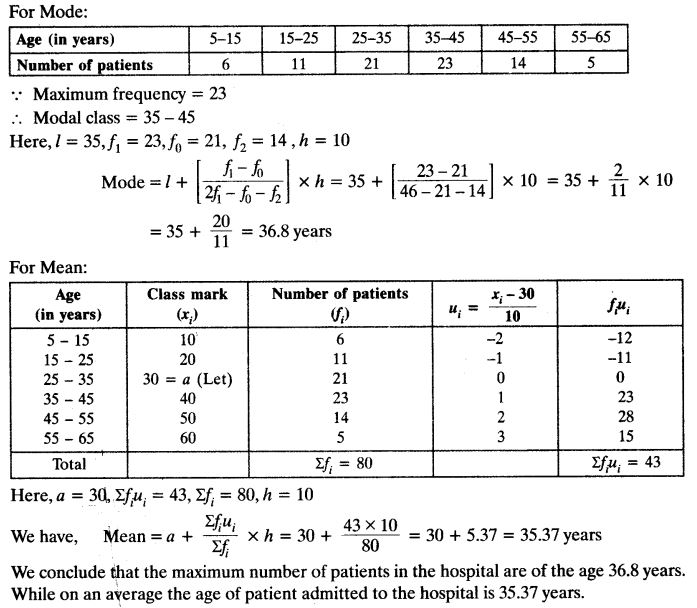Question 2.
The following data gives the information on the observed lifetimes (in hours) of 225 electrical components:Determine the modal lifetimes of the components.
Solution: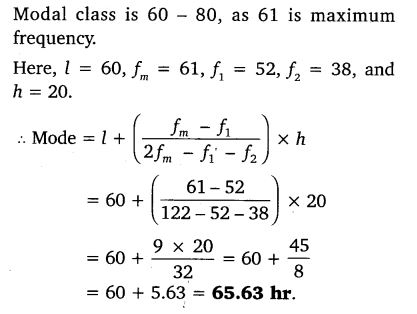Question 3.
The following data gives the distribution of total monthly household expenditure of 200 families of a village. Find the modal monthly expenditure of the families. Also, find the mean monthly expenditure:Solution: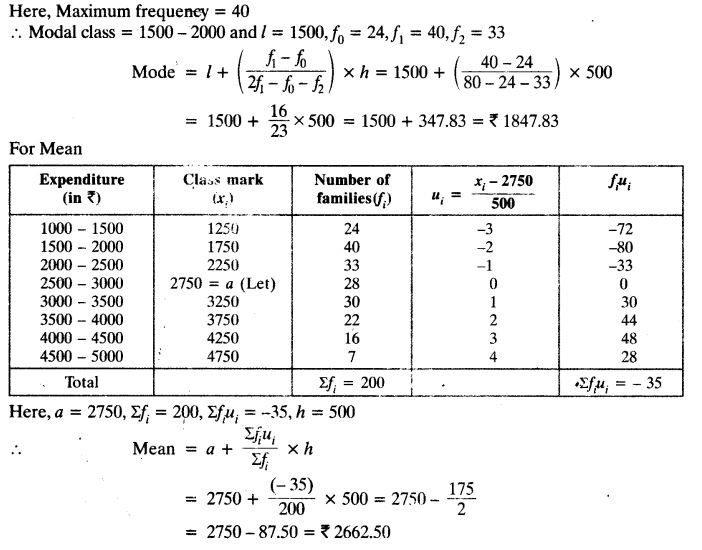Question 4.
The following distribution gives the state-wise teacher- student ratio in higher secondary schools of India. Find the mode and mean of this data. Interpret the two measures.Solution: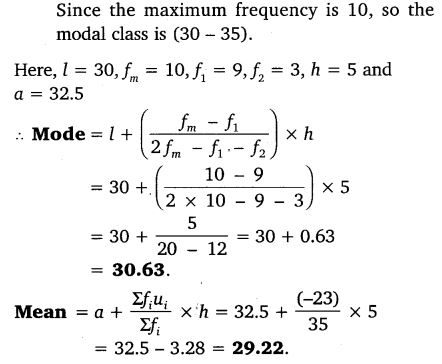Question 5.
The given distribution shows the number of runs scored by some top batsmen of the world in one-day international cricket matches.Find the mode of the data.
Solution: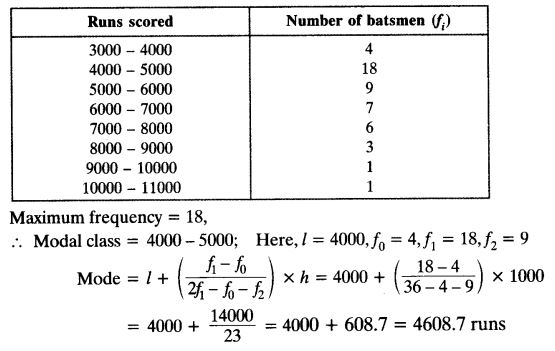Question 6.
A student noted the number of cars passing through a spot on a road for 100 periods each of 3 minutes and summarised it in the table given below. Find the mode of the data:Solution: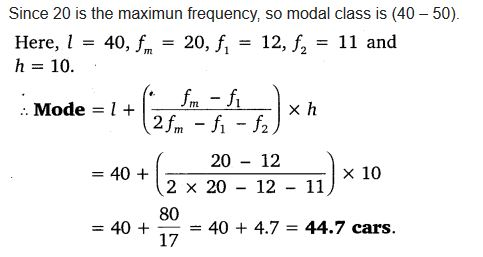error: Content is protected !!
+